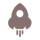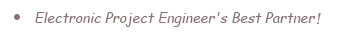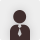New Customer?
Register
New here ? Getting \$50 coupon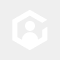T****yat
Linear Adjustable CC CV power supply Module
109 0 Dec 05.2021, 05:13:05

i would share to you my last project, the project is Linear Adjustable CC CV power supply Module, it can handle DC supply output up to 30VDC, and the output can be adjustable between 2.5VDC to 30VDC, the main components on this projects are OP AMP for measurement and shunt resistor for detecting current passing the load using Ohms's law where V= i x R. for example let's we have a look to picture below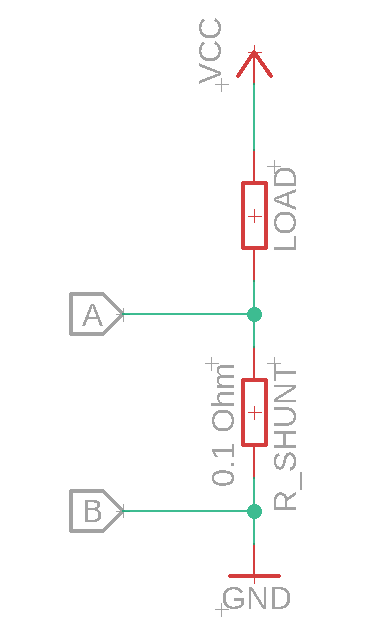when load was applied and current flowing trough Load and Shunt resistor (R_Shunt) there will be some voltage drop between point A and point B if the voltagae drop is 0.1V and R_SHUNT is 0.1 Ohm so the current can be found with simple formula

V = i x R

0.1V = i x 0.1Ohm

i = 0.1/0.1

i = 1 Amp in this case

here is full shcematic diagram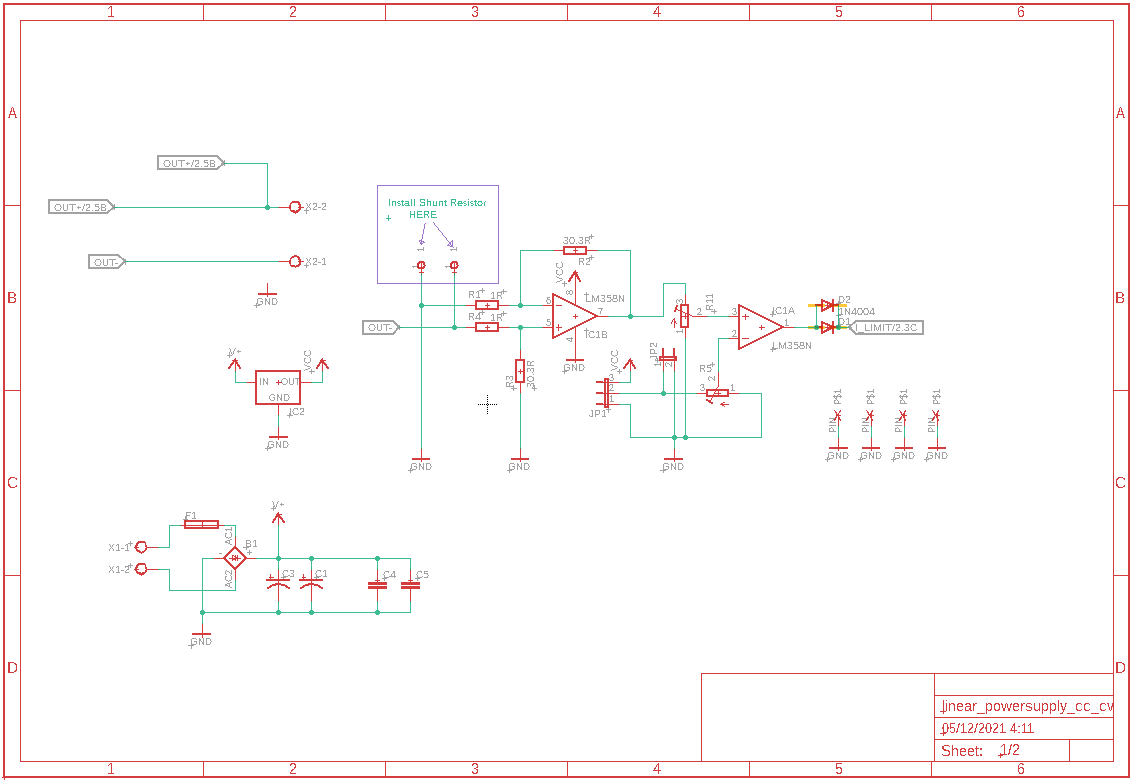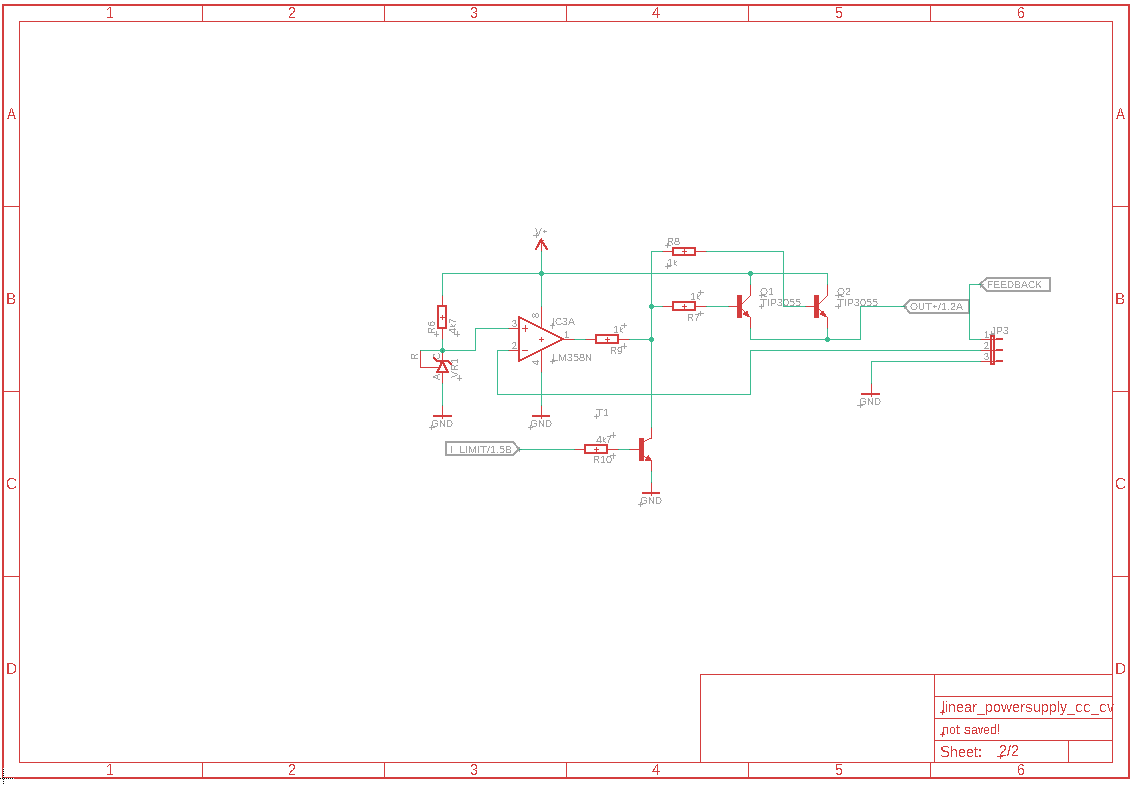Follow